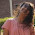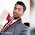### Why individual digits are read seperately after decimal point?

When we have to read a single whole number, say 2, we simply read it as "two". When we have to read three digit whole number, say 347, we read it as "three hundred and forty seven". It is quite natural to ask why digits after decimal point are read individually. For example, 0.347 will be read as "zero point three four seven" and not as "zero point three hundred and forty seven".

The reason for this is quite simple. Let us understand this with the help of an example :
Suppose we are given a set of three whole numbers, say 80, 080, 0080. Now if we try to read these numbers, we will read them all as "eighty". As there will be no change in their values even if we ignore to read the zero(s) before them, so we can read the whole number as a set of digits.

Now suppose we are given another set of three decimal numbers, say 0.80, 0.080, 0.0080. Now, let us try to read them as a set of digits, then we will read all the three numbers as "zero point eighty" ignoring all the zero(s) after decimal point. But all the three numbers have different values, so they can't be read same as their meanings are different. To solve this problem of mentioning where digits appear after the decimal point, we read individual digits after decimal seperately. Thus the above numbers will be read as "zero point eight zero", "zero point zero eight zero", "zero point zero zero eight zero" respectively.

1.I was very satisfied with this answer

2.3.Thats a grt answer...I never thought like that

4.To the point

5.The information you've provided here is fantastic because it provides a wealth of information that is really useful to me. Thank you for sharing that. Aeronatical Engineering Assignment Help

6.